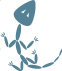Check-in [8a4059fa2f]

 ︙ ︙ ```31 32 33 34 35 36 37 38 39 40 41 42 43 44 45 46 47 48 49 ``` ```}; uint8_t TESTSHIFT=3; #define TESTMASK (((sizeof testsamples) / (sizeof testsamples))-1) #endif /* CIC filter state */ #define CIC2_R 8 #ifdef TWOSTEPCIC typedef int16_t CICREG; #else typedef int32_t CICREG; #endif CICREG s2_sum1 = 0; CICREG s2_comb1_1 = 0; CICREG s2_comb1_2 = 0; CICREG s2_sum2 = 0; CICREG s2_comb2_1 = 0; CICREG s2_comb2_2 = 0; CICREG s2_sum3 = 0; ``` ``` < < < < ``` ```31 32 33 34 35 36 37 38 39 40 41 42 43 44 45 ``` ```}; uint8_t TESTSHIFT=3; #define TESTMASK (((sizeof testsamples) / (sizeof testsamples))-1) #endif /* CIC filter state */ #define CIC2_R 8 typedef int32_t CICREG; CICREG s2_sum1 = 0; CICREG s2_comb1_1 = 0; CICREG s2_comb1_2 = 0; CICREG s2_sum2 = 0; CICREG s2_comb2_1 = 0; CICREG s2_comb2_2 = 0; CICREG s2_sum3 = 0; ``` ︙ ︙